Question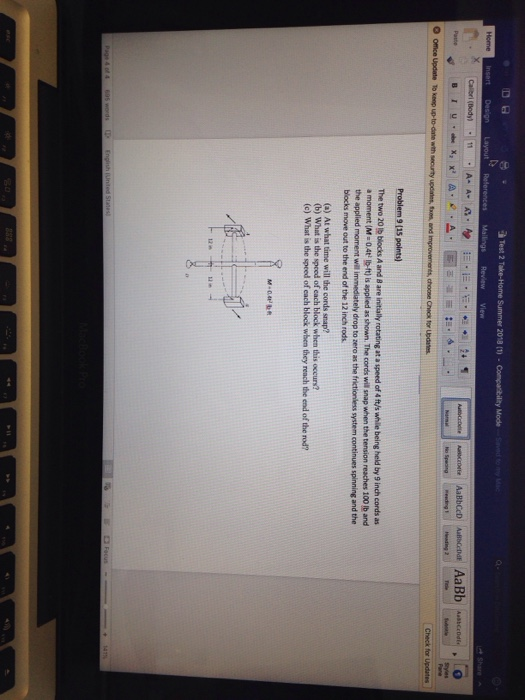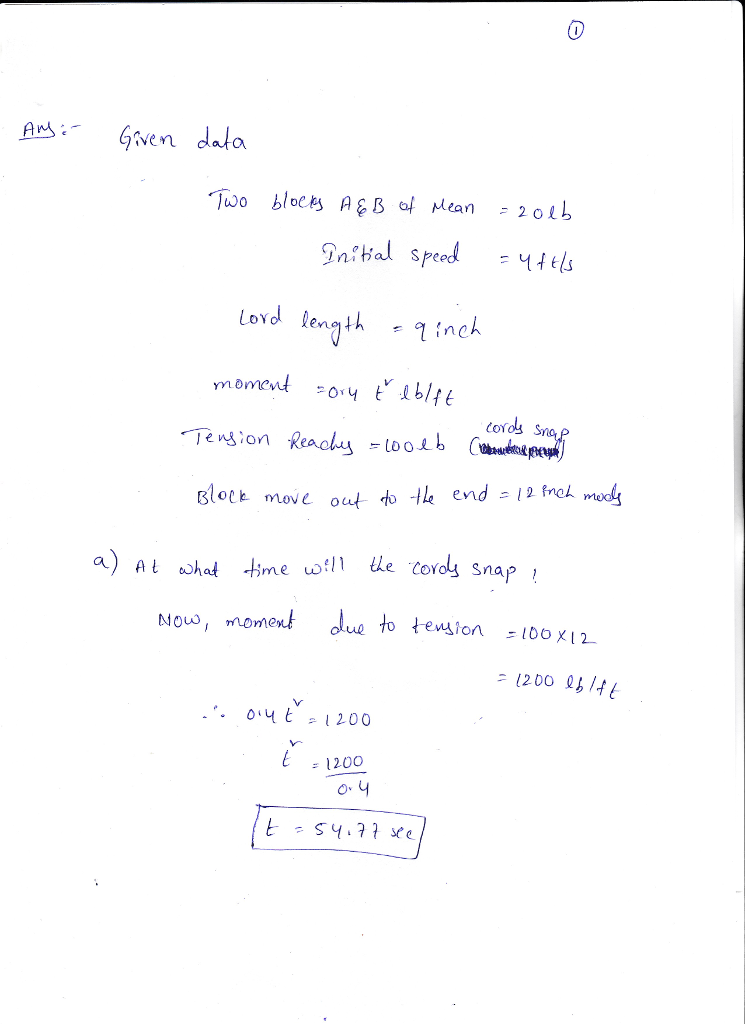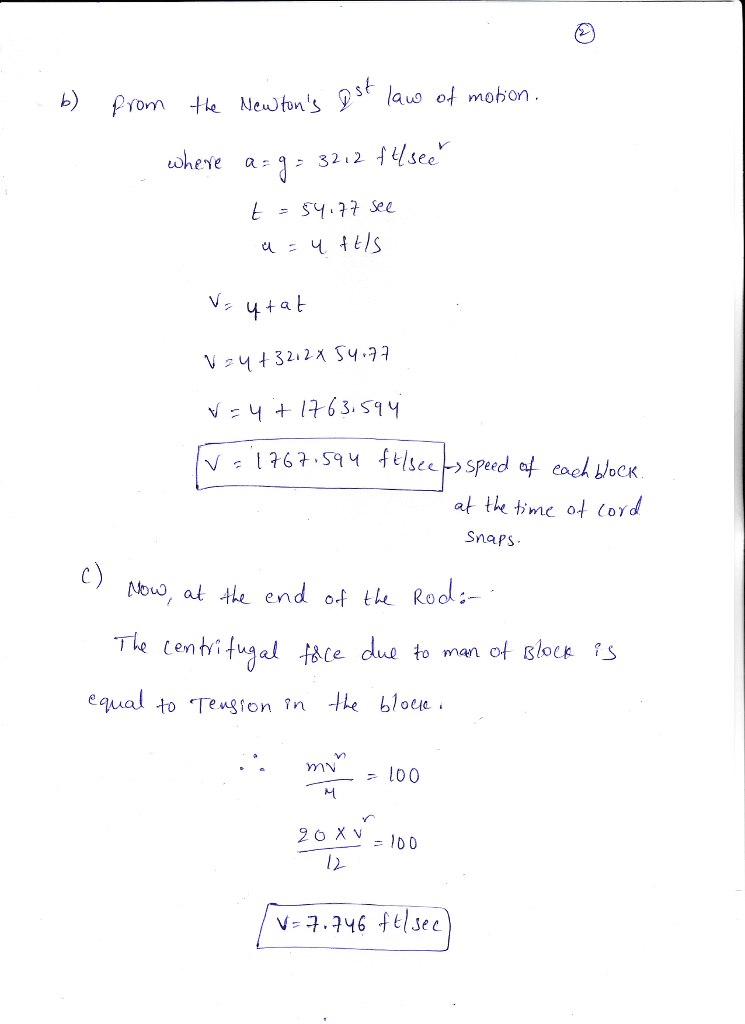#### Earn Coins

Coins can be redeemed for fabulous gifts.

Similar Homework Help Questions
• ### A long, uniform rod of mass 20.00 kg is pivoted at its top end. It is...

A long, uniform rod of mass 20.00 kg is pivoted at its top end. It is lifted a small angle and let go. When it reaches the very bottom of its swing, it is moving with angular speed ?o , and it has collision with a block of mass 3.000 kg that was originally at rest on a frictionless surface. After the collision the rod is seen to continue spinning in the same direction at 17.66 rad/s. As a result...

• ### 16) When the frictionless system shown above is accelerated by an applied force of magnitude F....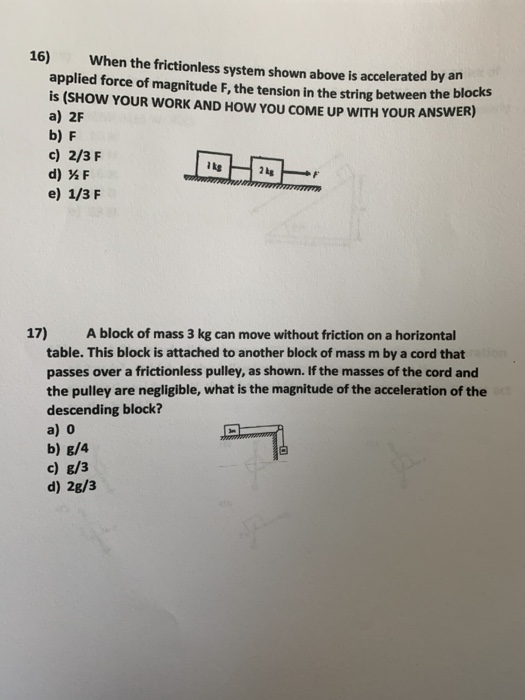16) When the frictionless system shown above is accelerated by an applied force of magnitude F. the tension in the string between the DIOCR is (SHOW YOUR WORK AND HOW YOU COME UP WITH YOUR ANSWER) a) 2F b) F c) 2/3 F d) F e) 1/3 F 17) A block of mass 3 kg can move without friction on a horizontal table. This block is attached to another block of mass m by a cord that passes over a...

• ### The 40kg uniform slender rod AB rests in the position shown when the constant couple moment of M ...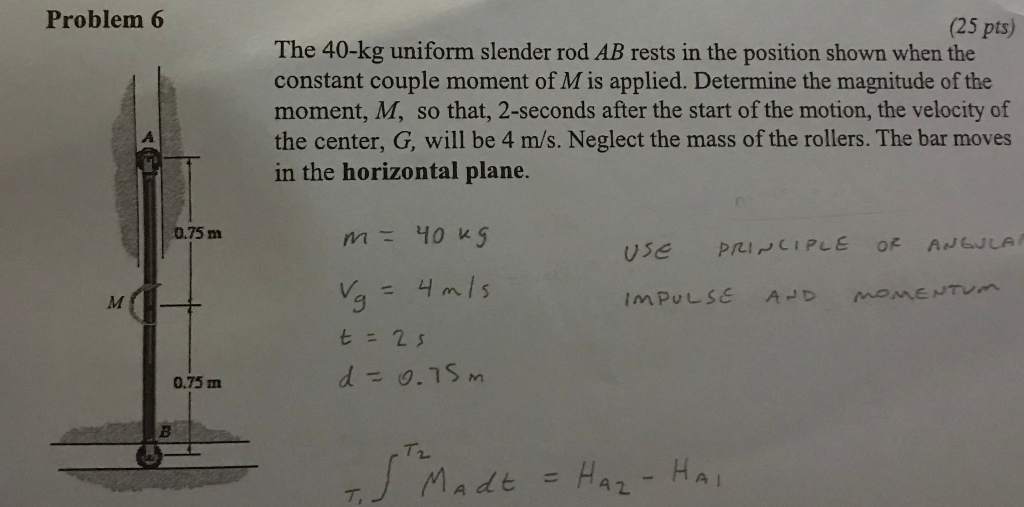The 40kg uniform slender rod AB rests in the position shown when the constant couple moment of M is applied. Determine the magnitude of the moment M, so that 2 seconds after the start of the motion, the velocity of the center, G, will be 4 m/s. Neglect the mass of the rollers. The bar moves in the horizontal plane. Problem 6 (25 pts) The 40-kg uniform slender rod AB rests in the position shown when the constant couple moment...

• ### This is the diagram that was provided. 3. It can be shown that the rotational inertia (moment of inertia) for a uniform...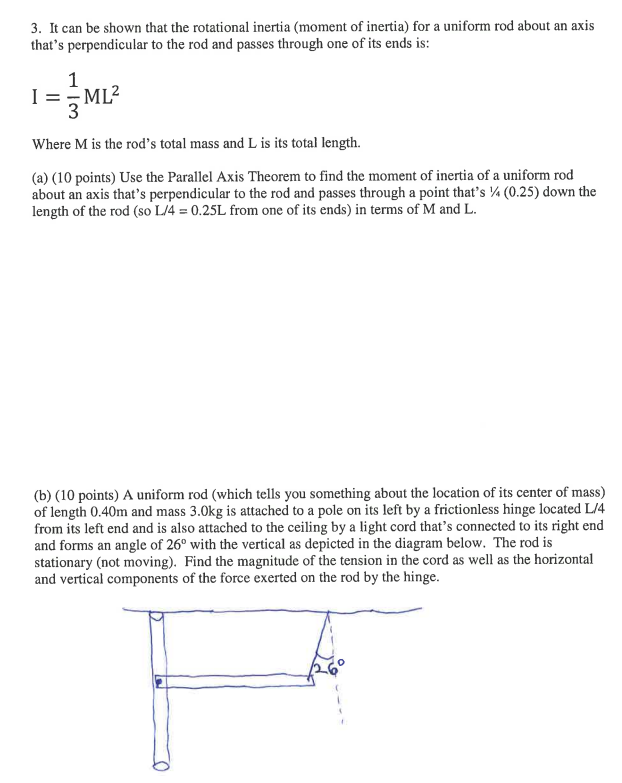This is the diagram that was provided. 3. It can be shown that the rotational inertia (moment of inertia) for a uniform rod about an axis that's perpendicular to the rod and passes through one of its ends is: Where M is the rod's total mass and L is its total length. (a) (10 points) Use the Parallel Axis Theorem to find the moment of inertia of a uniform rod about an axis that's perpendicular to the rod and passes...

• ### 4(12 points) A uniform rod of length L and mass M is attached at one end...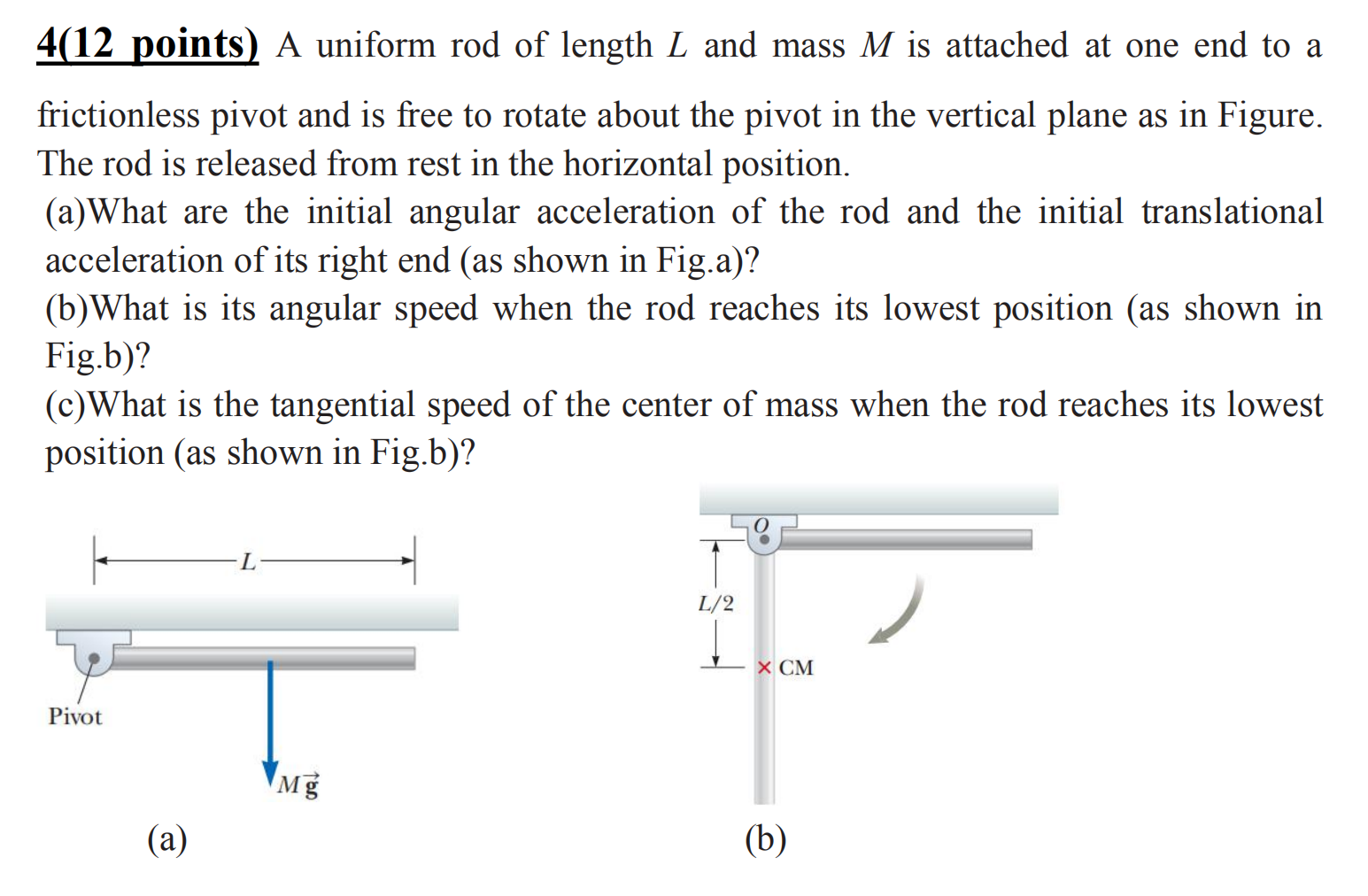4(12 points) A uniform rod of length L and mass M is attached at one end to a frictionless pivot and is free to rotate about the pivot in the vertical plane as in Figure. The rod is released from rest in the horizontal position. (a)What are the initial angular acceleration of the rod and the initial translational acceleration of its right end (as shown in Fig.a)? (b)What is its angular speed when the rod reaches its lowest position (as...

• ### The system shown in the figure below consists of a mass M = 3.3-kg block resting...

The system shown in the figure below consists of a mass M = 3.3-kg block resting on a frictionless horizontal ledge. This block is attached to a string that passes over a pulley, and the other end of the string is attached to a hanging m = 1.7-kg block. The pulley is a uniform disk of radius 8.0 cm and mass 0.60 kg. (a) What is the acceleration of each block? acceleration of M = 3.3 kg _____ m/s2 acceleration...

• ### Problem#6 A system comprising blocks, a light frictionless pulley, a frictionless incline, and connecting ropes is shown in the figure. The 9.0-kg block accelerates downward when the system...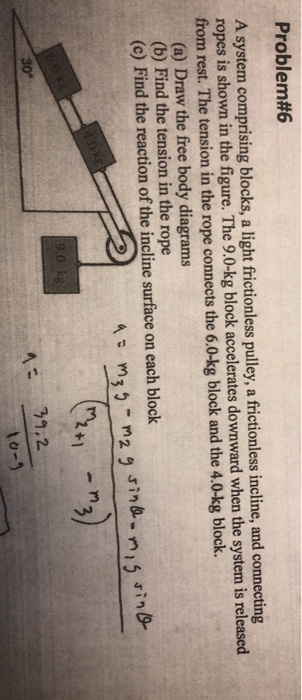Problem#6 A system comprising blocks, a light frictionless pulley, a frictionless incline, and connecting ropes is shown in the figure. The 9.0-kg block accelerates downward when the system is released from rest. The tension in the rope connects the 6.0-kg block and the 4.0-kg block. (a) Draw the free body diagrams (b) Find the tension in the rope (c) Find the reaction of the incline surface on each block rn +1 30° 10-9 Problem#6 A system comprising blocks, a light...

• ### genuinely have no idea how to approach this question ! (5 pts) You shoot a bullet at a vertical target that is a uniform mass M and moment of inertia l (1/2)MR. The target is free to rotate withou...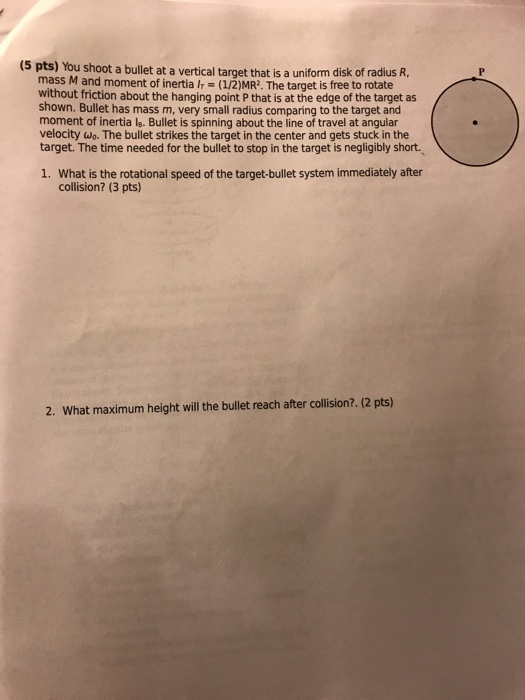genuinely have no idea how to approach this question ! (5 pts) You shoot a bullet at a vertical target that is a uniform mass M and moment of inertia l (1/2)MR. The target is free to rotate without friction about the hanging point P that is at the edge of the target as shown. Bullet has mass m, very small radius comparing to the target and moment of inertia le. Bullet is spinning about the line of travel at...

• ### 1.A playground merry-go-round has a radius of 3.0 m and a rotational inertia of 600 kgˑm2....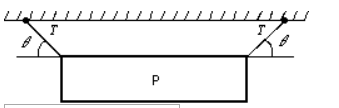1.A playground merry-go-round has a radius of 3.0 m and a rotational inertia of 600 kgˑm2. It is initially spinning at 0.80 rad/s when a 20 kg child crawls from the center to the rim. When the child reaches the rim the angular velocity of the merry-go-round in rad/s is: 2.A picture P of weight W = 40 N is hung by two strings as shown. The magnitude of the tension force of each string is T and θ=30°. The...

• ### If we change the axis to ?2 ball as shown. Determine the moment of inertia about...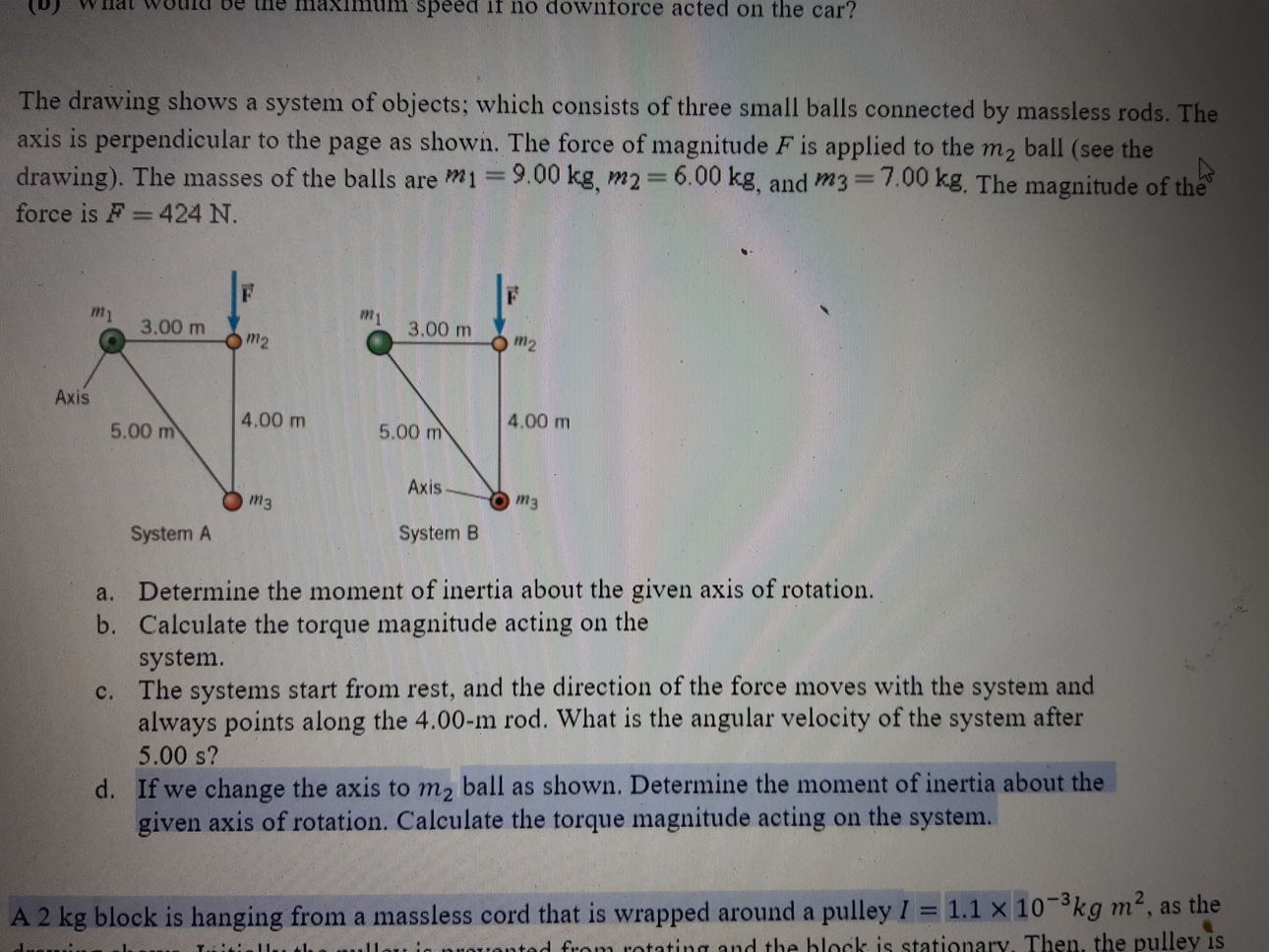If we change the axis to ?2 ball as shown. Determine the moment of inertia about the given axis of rotation. Calculate the torque magnitude acting on the system. nau be the speed if no downforce acted on the car? The drawing shows a system of objects, which consists of three small balls connected by massless rods. The axis is perpendicular to the page as shown. The force of magnitude F is applied to the m2 ball (see the drawing)....